# Accounting

## Quiz 11 : Financial Statement AnalysisStudy FlashcardsLooking for Accounting Homework Help?

## Quiz 11 :Financial Statement Analysis

Question TypeCalculate financial leverage measures The following information was available for the year ended December 31, 2016: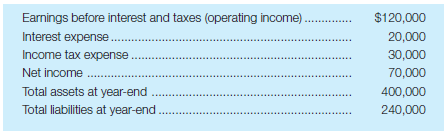Required: a. Calculate the debt ratio at December 31, 2016. b. Calculate the debt/equity ratio at December 31, 2016. c. Calculate the times interest earned for the year ended December 31, 2016.
Free
Essay

(a) Calculate debt ratio:
Debt ratio measure the amount of debt proportionate to total liabilities. Total liabilities include debt as well as equity. The debt ratio will be calculated by dividing the total liabilities with total liabilities and total stockholders. Use the following formula to calculate the debt ratio:Therefore, debt ratio is 0.6 or 60%.
(b) Calculate debt to equity ratio:
Debt to equity ratio measures the proportion of debt in the capital structure. The debt to equity ratio is calculated by dividing the total debt with the total stockholders' equity. Use the following formula to calculate the debt to equity ratio:.
Therefore, debt to equity ratio is 1.5 or 150%.
(c) Calculate times interest earned ratio:
Times interest earned measures a long term solvency of a company. This ratio indicates effectiveness of the company in meeting the interest expense over the obligation. The ratio is calculated by dividing the earnings before interest and taxes with the interest expense. Use the following formula to calculate the times interest earned ratio:Therefore, times interest earned is 6.

Tags
Choose question tagAnalytical case-comparative analysis of profitability and financial leverage measures The annual reports of the Coca-Cola Co. and PepsiCo, Inc., indicate the following for the year ended December 31, 2014 (amounts in millions):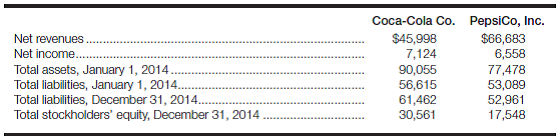Required: a. Calculate ROI and ROE for each company for 2014. (Hint: You will need to calculate some of the numbers used in the denominator of these ratios.)b. Based on the results of your ROI and ROE analysis in part a , do you believe that either firm uses financial leverage more effectively than the other? Explain your answer. (Hint: Compare the percentage differences between ROI and ROE for each firm. Is there a significant difference that would suggest that one firm uses leverage more effectively than the other?)c. Calculate the debt ratio and debt/equity ratio for each firm at the end of 2014. d. Compare the results of your analysis in part c to your expectations concerning the relative use of financial leverage in part b. Do the debt and debt/equity ratios calculated in part c make sense relative to your expectations? Explain your answer.
Free
Essay

(a) Calculate return on investment:
Return on investment  indicates the performance of the investment. It is very useful to compare with different investments and to select best investment. To calculate return on investment, the benefit from the investment i.e. income or return by the original cost of investment.For the company of CC,The company's ROI for the year 2014 are calculated by,Therefore, return on investment is 7.8%
For the company of PC,The company's ROI for the year 2014 are calculated byTherefore, return on investment is 8.9%.
(2) Calculate return on equity:
Return on equity shows the percentage of return earned on the common stock. It is calculated by dividing the net income with common stock.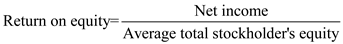For the company of CCThe company's ROE for the year 2014 is calculated by,Therefore, return on equity is 22.3%.
For the company of PC,The company's ROE for the year 2014 is calculated by:Therefore, return on equity is 31.3%.
(b)The ROI of CC Company is higher than the ROI of PC Company. However, the ROE of CC Company is lower than the ROE of PC Company. Therefore, the company of PC uses financial leverage more effectively than the other.
(c) Calculate debt and debt/equity ratio:
The debt ratio is calculated as under:Therefore, debt ratio is 66.8%.
The debt-equity ratio is calculated as under:
The debt-equity ratio is calculated as under:Therefore, debt/equity ratio is 2.01
For the PC Company,
The debt ratio is calculated as under:Therefore, Debt ratio is 75.1%.
The debt-equity ratio is calculated as under:Therefore, debt/equity ratio is 3.02.
(d)The debt ratio of CC Company is lower than the debt ratio of PC Company. Also, the debt/equity ratio of CC Company is lower than the debt/equity ratio of PC Company. It means that the PC Company uses higher debt. Both of them make sense relative to the expectations.

Tags
Choose question tagCalculate liquidity measures The following amounts were reported on the December 31, 2016, balance sheet: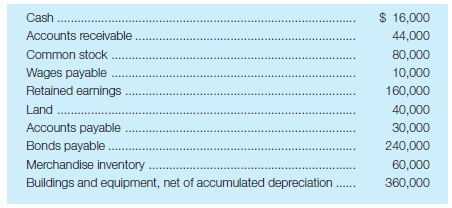Required: a. Calculate working capital at December 31, 2016. b. Calculate the current ratio at December 31, 2016. c. Calculate the acid-test ratio at December 31, 2016.
Free
Essay

(a) Calculate working capital at December 31, 2016:
Working capital measures the firm's ability to meet the current obligations as and when they are due. The working capital is the excess of current assets over current liabilities. The working capital is calculated by deducting the current liabilities from the current assets as shown below:Therefore, working capital is $80,000. (b) Calculate current ratio as at December 31, 2016: Current ratio is a measure to evaluate the liquidity to compare the time between firms. The current ratio is calculated by dividing the current assets with the current liabilities as shown below:Therefore, current ratio is 3. (c) Calculate acid-test ratio at December 31, 2016: Quick ratio is a conservative assessment of the firm's bill paying ability. It excludes inventories and other non-liquid current assets in determining the ratio. Use the following formula to calculate the quick ratio:Therefore, acid-test ratio is 1.5. Tags Choose question tagCalculate profitability measures The following information was available for the year ended December 31, 2016: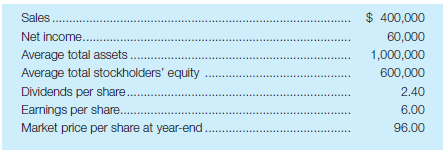Required: a. Calculate margin, turnover, and ROI for the year ended December 31, 2016. b. Calculate ROE for the year ended December 31, 2016. c. Calculate the price/earnings ratio for 2016. d. Calculate the dividend payout ratio for 2016. e. Calculate the dividend yield for 2016. Essay Answer: Tags Choose question tagIdentify information used in an investment decision Look forward to the day when you will have accumulated$5,000, and assume that you have decided to invest that hard-earned money in the common stock of a publicly owned corporation. What data about that company will you be most interested in, and how will you arrange those data so they are most meaningful to you? What information about the company will you want on a weekly basis, on a quarterly basis, and on an annual basis? How will you decide whether to sell, hold, or buy some more of the firm's stock?
Essay
Tags
Choose question tagRatio analysis-comprehensive problem Presented here are summarized data from the balance sheets and income statements of Wiper, Inc.: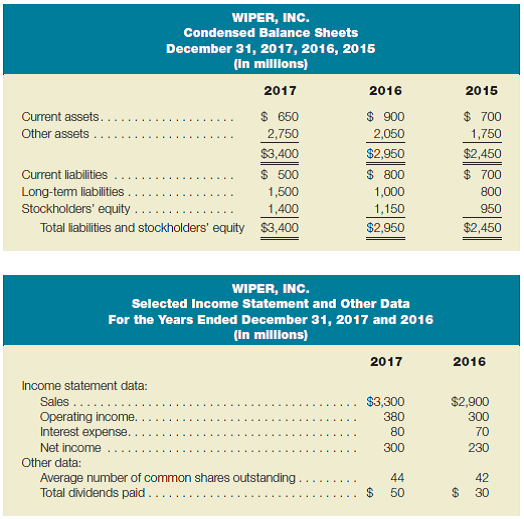Required: a. Calculate return on investment, based on net income and average total assets, for 2017 and 2016. Show both margin and turnover in your calculation. b. Calculate return on equity for 2017 and 2016. c. Calculate working capital and the current ratio for each of the past three years. d. Calculate earnings per share for 2017 and 2016. e. If Wiper's stock had a price/earnings ratio of 14 at the end of 2017, what was the market price of the stock? f. Calculate the cash dividend per share for 2017 and the dividend yield based on the market price calculated in part e. g. Calculate the dividend payout ratio for 2017. h. Assume that accounts receivable at December 31, 2017, totaled $310 million. Calculate the number of days' sales in receivables at that date. i. Calculate Wiper's debt ratio and debt/equity ratio at December 31, 2017 and 2016. j. Calculate the times interest earned ratio for 2017 and 2016. k. Review the results of these calculations, evaluate the profitability and liquidity of this company, and state your opinion about its suitability as an investment for a young, single professional with funds to invest in common stock. Essay Answer: Tags Choose question tagObtain an annual report and discuss information sources If your library has a common stock investment advisory service such as Moody's Handbook of Common Stocks or online access to an investment advisory service such as Value Line Research Center: Historical Reports, use one of those sources to locate a report about a company you have heard about or in which you have an interest. Alternatively, visit a brokerage firm office and ask for a report from one of the above sources or a report prepared by the brokerage firm's research division. Review the report and notice the analytical data that it contains. What other data besides those in the report would you like to obtain? Why do you want these other data? How would you get them? Essay Answer: Tags Choose question tagEffect of transactions on liquidity measures Selected balance sheet accounts for Tibbetts Company on September 30, 2016, are as follows: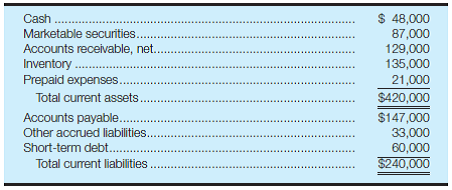Required: a. Calculate the working capital, current ratio, and acid-test ratio for Tibbetts Company as of September 30, 2016. b. Summarized here are the transactions/events that took place during the fiscal year ended September 30, 2017. Indicate the effect of each item on Tibbetts Company's working capital, current ratio, and acid-test ratio. Use + for increase, ? for decrease, and (NE) for no effect. (Hint: It may be helpful to use the horizontal model or to record the journal entry(ies) for each item before considering the effects on liquidity measures.)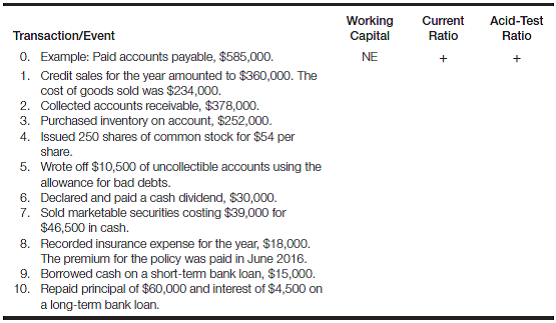Essay Answer: Tags Choose question tagCapstone analytical review of Chapters 9-11. Calculate selected financial ratios and explain financial reporting issues (Note: Please refer to Case 4.30 on pages 132 - 133 for the financial statement data needed for the analysis of this case. You should also review the solution to Case 4.30, provided by your instructor, before attempting to complete this case.)Required: a. Case 4.30 presents the 2016 income statement and balance sheet for Gerrard Construction Co. What other financial statements are required? What information would these statements communicate that could not be determined by reviewing only the income statement and balance sheet? b. Briefly describe the note disclosures that should be provided by Gerrard Construction Co., and explain why note disclosures are considered an integral part of the financial statements. c. Assume that the balance of "Accounts Receivable, net" at December 31, 2015, was$16,400. Calculate the following activity measures for Gerrard Construction Co. for the year ended December 31, 2016: 1. Accounts receivable turnover. 2. Number of days' sales in accounts receivable. d. Calculate the following financial leverage measures for Gerrard Construction Co. at December 31, 2016: 1. Debt ratio. 2. Debt/equity ratio. e. Gerrard Construction Co. wishes to lease some new earthmoving equipment from Caterpillar on a long-term basis. What impact (increase, decrease, or no effect) would a capital lease of $8 million have on the company's debt ratio and debt/equity ratio? (Note that these items were computed in part d and do not need to be recomputed for this requirement.)f. Review the answer to Case 4.30 part i at this time. Assume that Gerrard Construction Co. had 4,800,000 shares of$1 par value common stock outstanding throughout 2016, and that the market price per share of common stock at December 31, 2016, was $18.75. Calculate the following profitability measures for the year ended December 31, 2016: 1. Earnings per share of common stock. 2. Price/earnings ratio. 3. Dividend yield. 4. Dividend payout ratio. Reference Case 4.30: Capstone analytical review of Chapters 2-4. Calculate liquidity and profitability measures and explain various financial statement relationships for an excavation contractor Gerrard Construction Co. is an excavation contractor. The following summarized data (in thousands) are taken from the December 31, 2016, financial statements: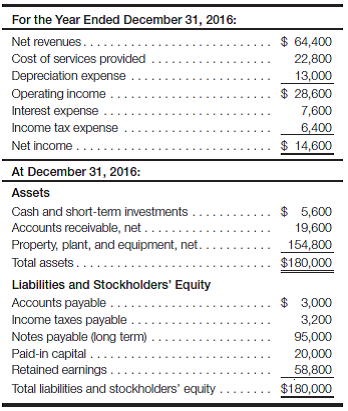At December 31, 2015, total assets were$164,000 and total stockholders' equity was $65,200. There were no changes in notes payable or paid-in capital during 2016. Required: a. The cost of services provided amount includes all operating expenses (selling, general, and administrative expenses) except depreciation expense. What do you suppose the primary reason was for management to separate depreciation from other operating expenses? From a conceptual point of view, should depreciation be considered a "cost" of providing services? b. Why do you suppose the amounts of depreciation expense and interest expense are so high for Gerrard Construction Co.? To which specific balance sheet accounts should a financial analyst relate these expenses? c. Calculate the company's average income tax rate. (Hint: You must first determine the earnings before taxes.)d. Explain why the amount of income tax expense is different from the amount of income taxes payable. e. Calculate the amount of total current assets. Why do you suppose this amount is so low, relative to total assets? f. Why doesn't the company have a Merchandise Inventory account? g. Calculate the amount of working capital and the current ratio at December 31, 2016. Assess the company's overall liquidity. h. Calculate ROI (including margin and turnover) and ROE for the year ended December 31, 2016. Assess the company's overall profitability. What additional information would you like to have to increase the validity of this assessment? i. Calculate the amount of dividends declared and paid during the year ended December 31, 2016. (Hint: Do a T-account analysis of retained earnings.) Essay Answer: Tags Choose question tagPrepare a common size balance sheet, 2013 Refer to the consolidated balance sheets of the Campbell Soup Company annual report in the appendix. Required: Prepare a common size balance sheet at July 28, 2013, using the following captions: Total current assets Plant assets, net of depreciation Goodwill Total other long-term assets Total assets Total current liabilities Total long-term liabilities (including deferred taxes)Total equity Total liabilities and equity Essay Answer: Tags Choose question tagCalculate activity measures The following information was available for the year ended December 31, 2016: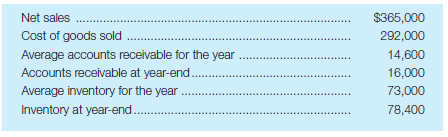Required: a. Calculate the inventory turnover for 2016. b. Calculate the number of days' sales in inventory for 2016, using year-end inventories. c. Calculate the accounts receivable turnover for 2016. d. Calculate the number of days' sales in accounts receivable for 2016, using year-end accounts receivable. Essay Answer: Tags Choose question tagFocus company-comprehensive financial statement analysis In Exercise 1.1, you were asked to obtain the most recent annual report of a company that you were interested in reviewing throughout this term. Required: Liquidity: a. Calculate working capital, the current ratio, and the acid-test ratio as of the most recent balance sheet date. b. Based on your calculations in part a, assess the company's overall liquidity position. Explain which ratios indicate particular strengths and/or weaknesses within the company. Assume the following industry averages: current ratio = 2.0; acid-test ratio = 1.6. c. Explain how working capital and the current ratio are related. Would you expect a company with a large amount of working capital to always have a high current ratio? Profitability: d. Calculate ROI, showing margin and turnover, for the most recent year. e. Calculate ROE for the most recent year. f. Calculate the price/earnings ratio for the most recent year, using the company's year-end market price per share of common stock in the numerator and diluted earnings per share in the denominator. g. Calculate the dividend payout and dividend yield ratios for the most recent year. h. Based on the results of your calculations in parts d, e, and f, assess the company's overall profitability. Explain which ratios indicate particular strengths and/or weaknesses within the company. Assume the following industry averages: ROI = 15%; margin = 10%; turnover = 1.5; ROE = 20%; price/earnings = 14.0; dividend payout = 40%; dividend yield = 5%. i. As an investor in this company's stock, would you be pleased with this year's dividend yield? How would your dividend yield "expectations" change, if at all, if the company's ROI was 5% higher? Explain. Financial leverage: j. Calculate the debt ratio and the debt/equity ratio as of the most recent balance sheet date. k. Based on the results of your calculations in part j, assess the company's overall leverage position. What would you estimate the industry averages to be for the debt ratio and debt/equity ratio? Explain. l. Explain the relationship between ROI and ROE, and the concept of financial leverage. Would you expect the percentage difference between ROI and ROE to be high or low for a firm that makes substantial use of financial leverage? Activity measures: m. Calculate the accounts receivable turnover and number of days' sales in accounts receivable (based on a 365-day year) for the most recent year. n. Based on your analysis in part m, do you believe that the company is doing an effective job at managing accounts receivable? What would you estimate the industry averages to be for the accounts receivable turnover and number of days' sales in accounts receivable? Explain. o. Calculate the inventory turnover and number of days' sales in inventory (based on a 365-day year) for the most recent year. p. Based on your analysis in part o, to what extent does the company need to be concerned about its inventory management policies? In assessing the inventory management policies, would you be more interested in knowing current ratio or acid-test ratio information? Explain. Overall assessment: q. Assume that you have$5,000 that you would like to invest in a single company. Evaluate the common stock of your focus company as a potential investment. From the data available in your focus company's financial statements, identify the five most important criteria that you would use to make your investment decision, and explain why each is important. Reference Exercise 1.1: Obtain an annual report Throughout this course, you will be asked to relate the material being studied to actual financial statements. After you complete this course, you will be able to use an organization's financial statements to make decisions and informed judgments about that organization. The purpose of this assignment is to provide the experience of obtaining a company's annual report. You may want to refer to the financial statements in the report during the rest of the course. Required: Obtain the most recently issued annual report of a publicly owned manufacturing or merchandising corporation of your choice. Do not select a bank, insurance company, financial institution, or public utility. It would be appropriate to select a firm that you know something about or have an interest in. Type firmname.com or use a search engine to locate your chosen company's website and then scan your firm's home page for information about annual report ordering. If you don't see a direct link to Investor Relations or Investors on the home page, look for links such as Our Company, About Us, or Site Map that may lead you to SEC Filings, Financial Information, or Annual Reports. Most companies allow you to save or print an Adobe Acrobat version of their annual reports.
Essay
Tags
Choose question tagEffect of transactions on various financial ratios Indicate the effect that each transaction/event listed here will have on the financial ratio listed opposite it, and provide an explanation for your answer. Use + for increase, ? for decrease, and (NE) for no effect. Assume that current assets exceed current liabilities in all cases, both before and after the transaction/event.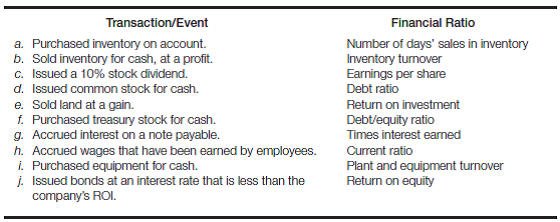Essay
Tags
Choose question tagReview problem-understanding liquidity measures Assume that the current ratio for Arch Company is 2.0, its acid-test ratio is 1.5, and its working capital is $900,000. Answer each of the following questions independently, always referring to the original information. a. How much does the firm have in current liabilities? b. If the only current assets shown on the balance sheet for Arch Company are Cash, Accounts Receivable, and Merchandise Inventory, how much does the firm have in Merchandise Inventory? c. If the firm collects an account receivable of$300,000, what will its new current ratio and working capital be? d. If the firm pays an account payable of $300,000, what will its new current ratio and working capital be? e. If the firm sells inventory that was purchased for$150,000 at a cash price of $180,000, what will its new acid-test ratio be? Essay Answer: Tags Choose question tagPrepare a common size balance sheet, 2014 Solve the requirements of Problem 11.9 for the year ended August 3, 2014. Reference Problem 11.9: Prepare a common size balance sheet, 2013 Refer to the consolidated balance sheets of the Campbell Soup Company annual report in the appendix. Required: Prepare a common size balance sheet at July 28, 2013, using the following captions: Total current assets Plant assets, net of depreciation Goodwill Total other long-term assets Total assets Total current liabilities Total long-term liabilities (including deferred taxes)Total equity Total liabilities and equity Essay Answer: Tags Choose question tagRatio analysis-comprehensive problem, 2014 data This problem is based on the 2014 annual report of Campbell Soup Company in the appendix. Required: a. Compute the following profitability measures for the year ended August 3, 2014: 1. Return on investment, based on net earnings (perform a DuPont analysis). 2. Return on equity, based on net earnings and total equity. 3. Price/earnings ratio. Use$41.96 as the year-end market price. 4. Dividend yield. 5. Dividend payout ratio. b. Compute the following liquidity measures at August 3, 2014: 1. Working capital. 2. Current ratio. 3. Acid-test ratio. c. Compute the following activity measures for the year ended August 3, 2014: 1. Number of days' sales in accounts receivable, based on a 365-day year. 2. Number of days' sales in inventory, based on a 365-day year. 3. Accounts receivable turnover. 4. Inventory turnover. 5. Turnover of net property, plant, and equipment. d. Compute the following financial leverage measures at August 3, 2014: 1. Debt ratio. 2. Debt/equity ratio. e. Compute the following physical measures of Campbell's profitability at August 3, 2014: 1. Net sales per employee. 2. Operating income per employee. (Note: In a page not reproduced in the appendix, Campbell's 2014 annual report disclosed that on August 3, 2014, the company had approximately 19,400 employees.)
Essay
Tags
Choose question tagEffect of transactions on various financial ratios Indicate the effect that each transaction/event listed here will have on the financial ratio listed opposite it, and provide an explanation for your answer. Use + for increase, ? for decrease, and (NE) for no effect. Assume that current assets exceed current liabilities in all cases, both before and after the transaction/event.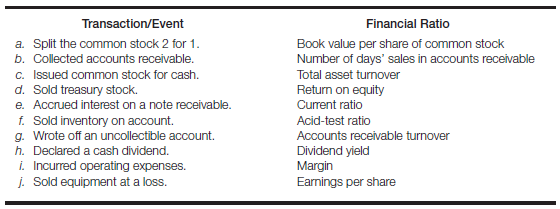EssayAnalytical case-complete an income statement and balance sheet using financial ratio data Partially completed financial statements for Whittaker, Inc., follow: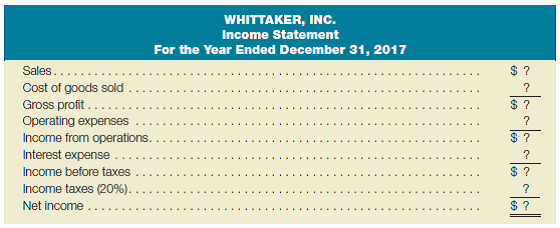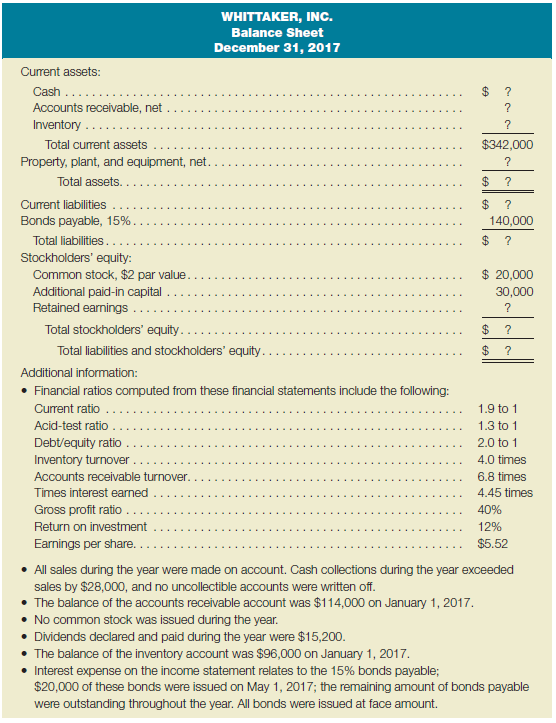Required: a. Complete the income statement and balance sheet for Whittaker, Inc. Show how each amount was determined. b. After completing part a, use your answers to recompute each of the financial ratios provided as additional information.